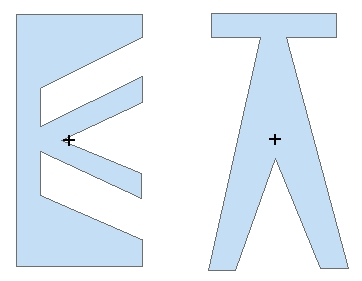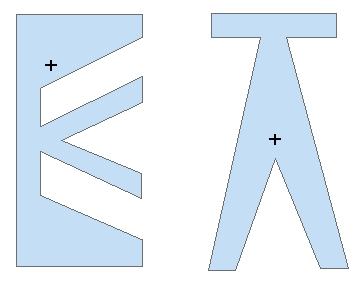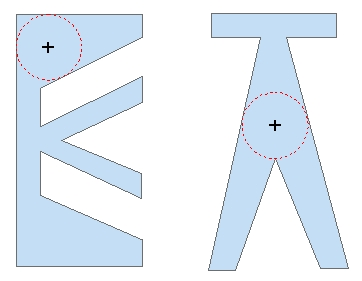Go to ET GeoWizards 12.x User Guide

Polygon To Point

Go to ToolBox Implementation

Converts a polygon data set to a point feature class

Inputs:

• A polygon feature layer
• Conversion option
• Vertices - the vertices of all polygons will be converted to points.
• Labels - the Label point is always located inside the polygon.  The algorithm makes sure that the point is not close to the boundary of the polygon. Points created using this algorithm are suitable for spatial transfer of attributes (See Smooth Polygons and Generalize Polygons functions).
• Centers - the Center points represent the centroid of a polygon. Therefore sometimes they might be located outside of the polygon
• Centers Inside - points representing the centroids of the polygons. If the centroid occurs outside of the polygon, the point is moved to be in the polygon.
• Deepest Point - a single point per polygon is created - the inside point farthest from the polygon boundary. The distance from the polygon boundary is stored in the ET_Depth field of the point attribute table
• More options
• Remove Duplicate Points - the duplicate points created from the vertices of two adjacent polygons will be represented by one point. Note that if this option is used the attempt to convert back these points to polygons will produce incorrect result
• Calculate point Position along boundaries
• If used  the [ET_Order] field will be populated with the relative location of the vertex (0 to 1) from the start of the polygon boundary.
• If not used, the [ET_Order] field will be populated with the order of the vertex in the polygon ring (from 0 to number of vertices)
• Preserve Z(M) available only if the input feature class is of PolygonZ(M) type. If selected, the result will be of PointZ(M) type, otherwise the result will be of  plain points (no Z or M values)

Outputs:

• New point feature class.
• All the original attributes of the polygons are transferred to the point attribute table
• new fields are added to the point attribute table
• [ET_Order] - the position of the point along the polygon's boundary. The value can be from 0 to 1 (if the Calculate point Position option is used) or from 0 to number of vertices (if not).  The value of this attribute can be used if the polygons have to be recreated from these points. - only if "Vertices" conversion option is used
• [ET_IDP] - the FID of original polygons. The values can be used to link the points back to the polygons.
• [ET_IDR] - this is a unique number identifying each ring of the polygons. If a polygon with FID = 356 has 3 rings, the corresponding points will have values in this fields 356_0, 356_1 and 356_2. This field can be used to recreate the polygons from the points without loosing the rings. - only if "Vertices" conversion option is used
• [ET_X] - the X coordinates of the resulting points
• [ET_Y] - the Y coordinates of the resulting points
• [ET_Z] - if the input feature class is of PolygonZ(M) type.
• [ET_M] - if the input feature class is of PolygonZ(M) type.
• [ET_DEPTH] - the distance from the deepest point to the polygon boundary. - only if "Deepest Point" conversion option is used

Notes :

• See above for the use of the "Remove duplicate points" option
• The functionality of the PolygonZ(M) To Point function available in the pre 11.0 versions is entirely included in this function.

Examples:

 Input Dataset Result Vertices option Result Labels optionResult Center option Result Center in option Result Deepest Point optionCommand line syntax

ET_GPPolygonToPoints <input_dataset> <out_feature class> <Vertex | Center | Label> {remove_duplicates} {calc_point_pos} {keep_ZM}

Parameters

Part Description
<input_dataset> A Polygon feature class or feature layer
<out_feature class> A String - the full name of the output feature class (A feature class with the same full name should not exist)
<Vertex | Center | LabelCenterIn|DeepestPoint> Convert Option. A String -  the export option to be used. The available options are (Case sensitive)
• "Vertex" -  - the vertices of all polygons will be converted to points. If {remove_duplicates} is True  the duplicate points created from the vertices of two adjacent polygons will be represented by one point.
• "Label" - the Label point is always located inside the polygon.  The algorithm makes sure that the point is not close to the boundary of the polygon. Points created using this algorithm are suitable for spatial transfer of attributes (See Smooth Polygons and Generalize Polygons functions).
• "Center" - the Center points represent the centroid of a polygon. Therefore sometimes they might be located outside of the polygon
• "CenterIn" - points representing the centroids of the polygons. If the centroid occurs outside of the polygon, the point is moved to be in the polygon.
• "DeepestPoint - a single point per polygon - the inside point farthest from the polygon boundary.
{remove_duplicates} A Boolean  used only with Convert Option = "Vertex". If True the duplicate points representing coincident vertices of two or more adjacent polygons will be removed.
{calc_point_pos} A Boolean indicating whether the position of the points along the polygon boundary to be calculated (only if the "Vertex option is used)
{keep_ZM} A Boolean indicating whether the the output will be of Z(M) type (only if the input dataset is of Z(M) type)

Scripting syntax

ET_GPPolygonToPoints (input_dataset, out_feature class, convert_option, remove_duplicates,calc_point_pos, keep_ZM)

See the explanations above:
<> - required parameter
{} - optional parameter